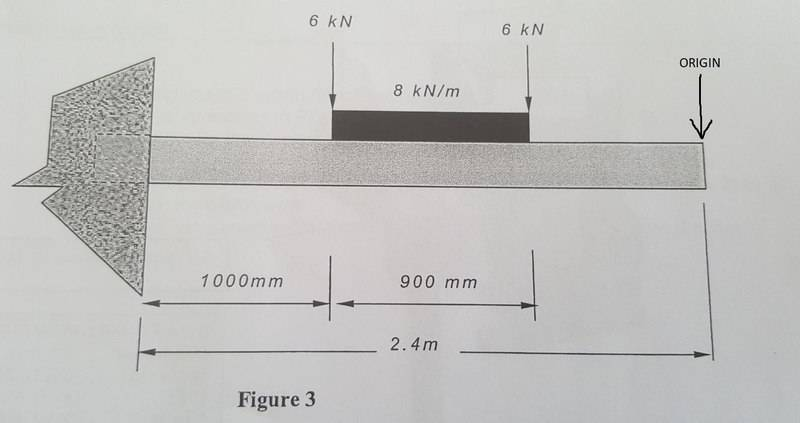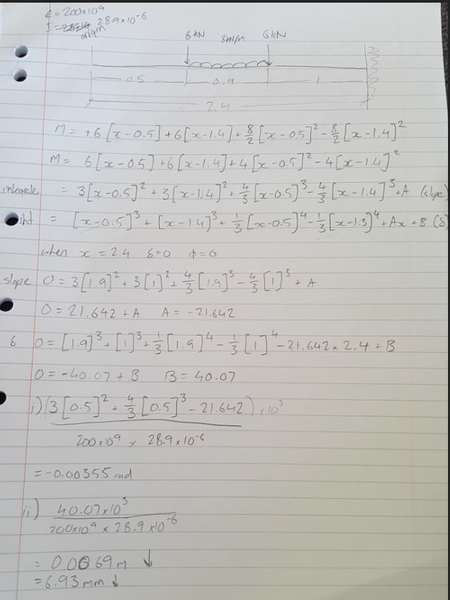# Using Macaulay's method of deflection on a cantilever beam

## Homework Statementi) Determine the slope of the beam 1m from the wall.
ii) Calculate the deflection at the free end of the beam.

## Homework Equations

Macauly's method of deflection: M = -RX + W[X-a]
Integrate once for slope
Integrate twice for deflection

## The Attempt at a Solution[/B]
It is my understanding that a positive deflection is downwards and a negative deflection is upwards.

I am getting a negative answer for the slope which would indicate an upwards slope?

When I use my initial equation to calculate the moment before integrating, I get the correct moment value (27.84kNm) so I feel like that is correct, Im worried that maybe some of the values in my equation should be negative which would change the value of my constants A and B.

Any help would be greatly appreciated.

#### Attachments

haruspex
Homework Helper
Gold Member
Your hand drawn diagram does not match the printed one. You have 0.5m to the first load where the printed diagram has 1m.

Also, shouldn't there be a torque and vertical force from the support in the expression for M?

Your hand drawn diagram does not match the printed one. You have 0.5m to the first load where the printed diagram has 1m.

Also, shouldn't there be a torque and vertical force from the support in the expression for M?

Hi, sorry ive drawn the beam out mirrored as I was trying to compare it to a similar one done in class. My diagram is shown with the fixed end on the right.

There is 19.2kN reaction force at the fixed end, I am just not sure whether this is incorporated into the equation.

haruspex
Homework Helper
Gold Member
My diagram is shown with the fixed end on the right.
Ok, so you are measuring x from the free end, but the question asks for the slope 1m from the wall, and you have substituted x=1.
Also, viewed from the free end, your loads will have clockwise moments, so should have negative torques. That is why you got a negative slope.

haruspex